# Physics - Electromagnetism - Cathode ray tubes explained using electric potential
Source

## Introduction

Hello it's a me again Drifter Programming!

Today we continue with Electromagnetism to get into an explanation of how Cathode ray tubes work, whose technology is used in oscilloscopes and older televisions and monitors.

So, without further do, let's get straight into it!

## Cathode ray tube explanation

The cathode ray tubes use an electron beam, that is also called an cathode ray, because it originates from the cathode (negativelly charged electrode or electricity conductor) of an vacuum light bulb.

The inside of such a tube has a very small pressure of 0.01Pa or less an so "simulates" a high vacuum of space. If it wasn't like that then the electrons would interact with the air molecules and so the beam would be scattered around.

An cathode ray tube is build like that:Source

To make the fluorescent screen "display" something the following needs to be done:

1. The cathode is heated up to a high temperature from the boiler and the electrons "evaporate" from the cathode surface.
2. The acceleration anode has a small cut in the center so that the electrons can pass through and also has a high positive potential of 1 up to 20kV to the cathode.
3. The potential difference of the anode and cathode creates an electric field that goes from the anode to the cathode (right to left).
4. The electric force that gets applied to the electrons (negative charge) goes in the opposite direction of the electric field and so to the right, which means that the electrons go through the small cut by forming a thin beam that after that continues on travelling with an constant velocity from the anode to the fluorescent screen.
5. When the electrons "hit" the screen, the area that is being hit fluoresces intensely and that way "displays" something.
6. To manage the number of electrons that should pass-through we use an control grid, which of course helps us adjust the brightness of the "hit" spot.
7. The electrons that leave the cathode have different directions and so to focus them into a single beam a focussing anode is being used.
8. To specify the actual point where the electrons should hit we use horizontal and vertical deflection plates.

Let's analyze the motion of the electrons...

For the mathematical equations I will use the tool quicklatex!

Using the conservation of energy we know that the sum of kinetic and potential energy is constant through-out the motion of the electron (electric forces are conservative) and so: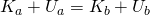Let's suppose that point a is the point where the electron is standing still (zero kinetic energy)

That way: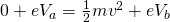where e of course is the charge of an electron.

Solving for the velocity (v) we end up with: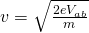where Vab is of course the potential difference of the anode to the cathode.

The mass and charge of an electron are of course constant and so the only thing that velocity and kinetic energy depend on is the potential difference V when inside of the electric field of the anode and cathode.

This means that the velocity that the electron has when pushing through the small cut of the anode can be calculated!

After leaving the anode and so the electric field of the anode and cathode the electron is now in the area of the vertical deflection plates.

When there is no electric field between those plates then the electron "continues it's course" and so hits the screen following the direction that it had when leaving the electric field (the gravity has a very small impact).

When there is an potential difference V between those plates, with the top one being positively charged, then there is an electric field with a downward direction (E = V/d) that applies a constant force eE upwards to the electron with an acceleration of: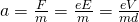where d is of course the distance of the plates.

The horizontal velocity of the electron stays the same and so an "vertical component" has now been created by causing the electron to move in a parabola trajectory.

Supposing the length of the plates as L the time taken of the electron to leave this area is: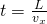where vx is the horizontal velocity of the electron.

Using this time we can now calculate the vertical velocity:The deviation angle with which the electrons exit this field is: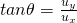Approximating, because L (length) << D (distance) we have:where we also can substitude ux and so get the final result:where V2 is the potential difference of the vertical deflection plates and V1 is the potential difference of the "first" electric field of the anode-cathode.

This final equation gives us the "distance" traveled vertically and so the vertical hit point location (somewhat). We can also see that the location again depends on a lot of constant and known things and so the location can be specified very easily.

After that the electron gets into the horizontal deflection plate area where the beam deviates in the horizontal direction, vertically across to the other "previous" direction.

I will not get into the math of that, cause it's similar...

You can see that coordinating the electron is not that difficult and we just have to adjust the electric fields of the deflection plates.

This kind of technology is mostly used in oscilloscopes. In televisions we have magnetic fields, which work similarly and that we will discuss in later articles!

Intro

Physics Introduction -> what is physics?, Models, Measuring

Vector Math and Operations -> Vector mathematics and operations (actually mathematical analysis, but I don't got into that before-hand :P)

Classical Mechanics

Velocity and acceleration in a rectlinear motion -> velocity, accelaration and averages of those

Rectlinear motion with constant accelaration and free falling -> const accelaration motion and free fall

Rectlinear motion with variable acceleration and velocity relativity -> integrations to calculate pos and velocity, relative velocity

Rectlinear motion exercises -> examples and tasks in rectlinear motion

Position, velocity and acceleration vectors in a plane motion -> position, velocity and accelaration in plane motion

Projectile motion as a plane motion -> missile/bullet motion as a plane motion

Smooth Circular motion -> smooth circular motion theory

Plane motion exercises -> examples and tasks in plane motions

Force and Newton's first law -> force, 1st law

Mass and Newton's second law -> mass, 2nd law

Newton's 3rd law and mass vs weight -> mass vs weight, 3rd law, friction

Applying Newton's Laws -> free-body diagram, point equilibrium and 2nd law applications

Contact forces and friction -> contact force, friction

Dynamics of Circular motion -> circular motion dynamics, applications

Object equilibrium and 2nd law application examples -> examples of object equilibrium and 2nd law applications

Contact force and friction examples -> exercises in force and friction

Circular dynamic and vertical circle motion examples -> exercises in circular dynamics

Electromagnetism

Getting into Electromagnetism -> electromagnetim, electric charge, conductors, insulators, quantization

Coulomb's law with examples -> Coulomb's law, superposition principle, Coulomb constant, how to solve problems, examples

Electric fields and field lines -> Electric fields, Solving problems around Electric fields and field lines

Electric dipoles -> Electric dipole, torque, potential and field

Electric charge and field Exercises -> examples in electric charges and fields

Electric flux and Gauss's law -> Electric flux, Gauss's law

Applications of Gauss's law (part 1) -> applying Gauss's law, Gauss applications

Applications of Gauss's law (part 2) -> more Gauss applications

Electric flux exercises -> examples in electric flux and Gauss's law

Electric potential energy -> explanation of work-energy, electric potential energy

Calculating electric potentials -> more stuff about potential energy, potential, calculating potentials

Millikan's Oil Drop Experiment -> Millikan's experiment, electronvolt

And this is actually it for today!

Next time we will get into examples/exercises of Electric potential.

I think I will split those exercises into two parts, just so that I can cover everything!

Bye!

H2
H3
H4
3 columns
2 columns
1 column
1 Comment